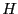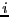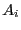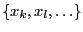Next: Implementation Up: Regularity of matrix with Previous: Regularity of matrix with   Contents

Mathematical background

Let assume that a parametric matrix has rows (or columns) in which some elements depend linearly on some of the unknowns. Let consider the setof interval matrices obtained as follows:
• for rowletbe the elements that depend linearly on the unknowns(called the linear unknowns)
• construct all possible rows for rowby fixing the linear unknowns either to their minimum or maximum
• construct the setby taking all possible combinations of all rows
It can be then shown that if all the matrices inhave their determinants of the same sign, then the set of parametric matrices does not include any singular matrix. This test is more powerful than the test proposed by Rohn (see section 7.4.2) as it takes more into account the structure of the parametric matrix.

Jean-Pierre Merlet 2012-12-20